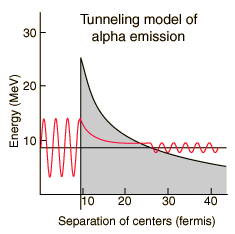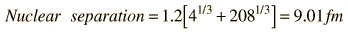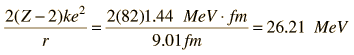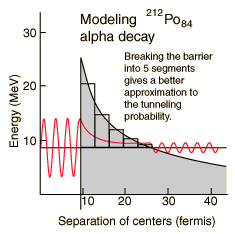Modeling Alpha Half-lifeA number of parameters must be calculated to model the barrier penetration which leads to alpha emission . The nuclear influence is assumed to stop sharply when the emitted alpha and the reduced nucleus are just touching each other. Using the nuclear radius relationship, this distance for polonium-212 isFor a nucleus with atomic mass A, the above relationship is used with A-4. For A = , then r = fermis. The height of the barrier at the above distance is calculated as the Coulomb potential of point charges. The charge of the remaining nucleus has been reduced by two by the alpha emission, so that barrier height for polonium-212 is:and for Z = it is MeV

The distance at which the Coulomb potential drops to the level of energy of the observed polonium-212 alpha isFor an alpha energy of MeV, the distance is fermi.

So the width of the polonium-212 barrier isand the width for the currently modeled nucleus is fermi.

In addition to the tunneling probability calculated below, the alpha emission rate depends upon how many times an alpha particle with this energy inside the nucleus will hit the walls. The velocity of the alpha can be calculated from

[Image_Link]imgnuc/atun5.png>

since an alpha at this energy is nonrelativistic. The frequency of hitting the walls is thenFor the currently modeled nucleus, the frequency is f = x 10^ /s.This model gives a halflife of 0.24 microseconds for polonium-212 compared to the experimental halflife of 0.3 microseconds. Not bad! But it must be admitted that this is a fortuitous example. Not all of them agree this well with just a five segment barrier approximation.

The tunneling probability is modeled here by breaking the barrier into five segments and multiplying the successive tunneling probabilities. The following table was constructed by dividing the barrier width into five equal segments with height equal to the midpoint height of the segment.

 Segment height Probability of tunneling x10^ x10^ x10^ x10^ x10^ Product ofprobabilities x10^

For a given alpha, the combined tunneling probability per second for emission is the product= x10^ /s

which gives half-life = x10^ s

 Discussion of polonium alpha decay Integration of the tunneling barrier
Index

References
Rohlf
Sec 7-4

Eisberg & Resnick
Sec 16-2

 HyperPhysics***** Nuclear R Nave
Go Back